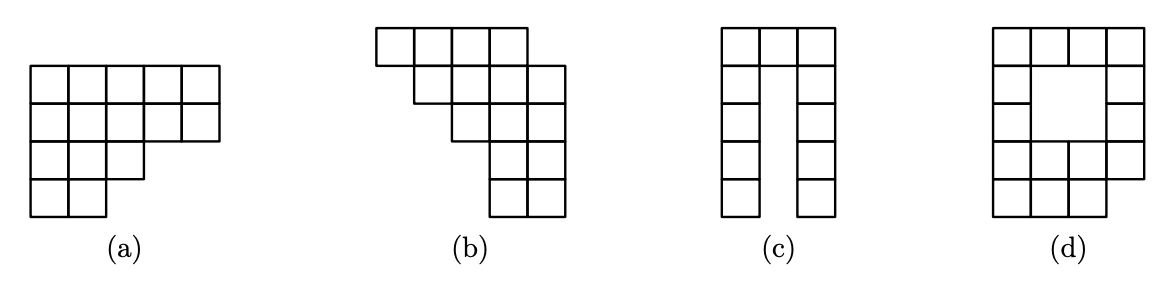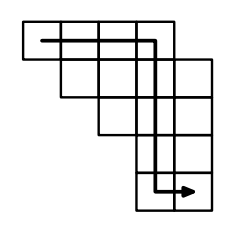시간 제한 메모리 제한 제출 정답 맞은 사람 정답 비율
2 초 512 MB 1 1 1 100.000%

## 문제

A polyomino is a connected set of unit squares on a square grid. The picture below shows 4 examples of polyominoes.Polyomino is called convex if its intersection with any vertical or horizontal line is a segment. The picture above shows two convex polyominoes (a) and (b) and two non-convex ones (c) and (d).

Two squares are called adjacent if they share a common side. It is easy to see that for any two squares of a convex polyomino it is possible to get from any square to any other one moving from a square to adjacent one and using only two directions. The picture below shows an example of such path for polyomino (b).For a convex polyomino $P$ let us define its jinxiety $J(P)$ as a minimal $k$ such that it is possible to get from any square to any other square by a path that uses two directions and makes at most $k$ turns. For example, the polyomino (a) has jinxiety of 1 and polyomino (b) has jinxiety of 2.

Given a convex polyomino you have to find its jinxiety.

## 입력

The input file contains multiple test cases.

Each test case contains two integers $h$ and $w$ --- the number of rows and columns in polyomino description, respectively ($1 \le h, w \le 2000$).

The following $h$ lines contain $w$ characters each and describe the polyomino.  Each character is either "." for an empty square, or "#" for a polyomino square. It is guaranteed that the described figure is a convex polyomino.

Input is followed by a line with $h = w = 0$. The total number of characters in all polyomino descriptions of the input file is at most $4\cdot10^6$. There are at most $40\,000$ tests.

## 출력

For each test case print one integer: the jinxiety of the polyomino in the input.

## 예제 입력 1

4 5
#####
#####
###..
##...
5 5
####.
.####
..###
...##
...##
0 0


## 예제 출력 1

1
2